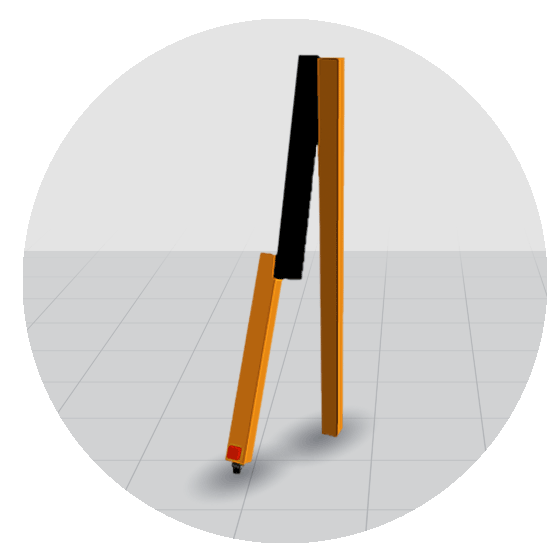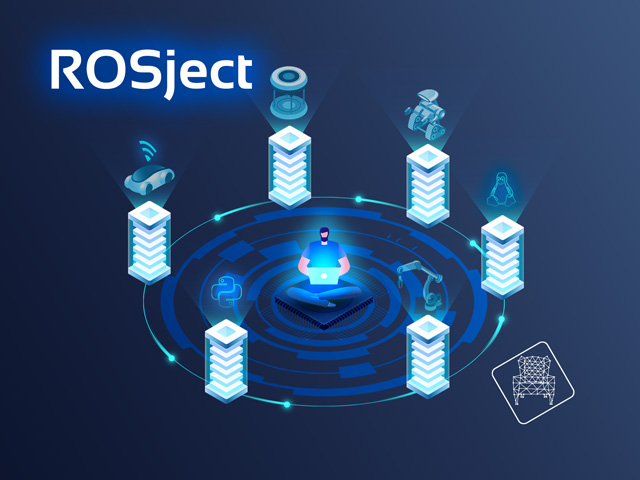# Robot Dynamics and Control Course - Python

Learn to develop dynamic models and intelligent control systems for simple robots.## Course Summary

Robot Dynamics is really important since it will give you a complete understanding not only of how the robots move (kinematics) but also WHY they move (dynamics). In this course, you will learn to develop the dynamics models of basic robotic systems, as well as create intelligent controllers for them.

## What you will learn

• How to solve the dynamics for the motion of rigid bodies in 3D space with the use of Newton's laws of motion
• How to model the dynamics of a simple robotic system and how to derive its equations of motion
• How to create a full state feedback controller to allow a robotic system to balance

## Course Overview

### Introduction

This unit presents the objectives of this course on dynamics for robot manipulators, and the learning methods and tools.

### Rigid Body Dynamics

In this Unit you will learn how to solve for the motion of rigid bodies in 3D space with the use of Newton's laws of motion.

### Dynamic Modeling

In this Unit you will learn how to model the dynamics of a simple robotic system and how to derive its equations of motion.

### Feedback Control

In this unit, we will create a full state feedback controller for allowing the robot to balance.

### Project. Ball Kicking

In this project you are going to program a simple dynamic controller for the RRBot arm that will move the robot for kicking a ball on the floor.

## Teachers

### Enric Cervera Mateu

Robotics and Computing teacher at the University Jaume I de Castelló,. Researcher on AI applied to social and educational robotics. Has collaborated in projects with hospitals on how to use robots for autistic child therapies.## Robots used

### RRBot robot## Learning Path

Basic Robotics Theory